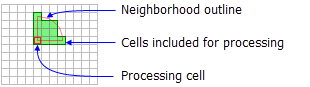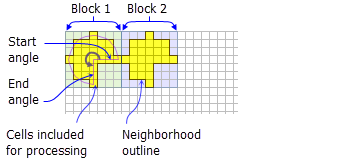# NbrWedge

Mit der Spatial Analyst-Lizenz verfügbar.

## Zusammenfassung

Defines a wedge neighborhood which is created by specifying a radius and two angles in either map units or number of cells.

## AbbildungNbrWedge neighborhood example for the FocalStatistics function (radius 3 cells, start angle 0, end angle 90).NbrWedge neighborhood example for the BlockStatistics function (radius = 3 cells, start angle = 0, end angle = 270).

## Auswertung

Tools that use neighborhood wedge object: Block Statistics, Focal Statistics, Point Statistics, and Point Density.

##### Lizenz:

This class is also available if you have an Image Analyst extension license, but only for the Focal Statistics tool.

A wedge is specified by a radius, a startAngle, an endAngle, and the units. The wedge extends counterclockwise from the starting angle to the ending angle. Angles are specified in arithmetic degrees (counterclockwise from the positive x-axis). Negative angles may be used.

The radius is identified in cells or map units, measured perpendicular to the x- or y-axis. When the radius is specified in map units, it is converted to a radius in cell units. The resulting radius in cell units produces an area that most closely represents the area calculated by using the original radius in map units. Any cell center encompassed by the wedge will be included in the processing of the neighborhood.

## Syntax

`NbrWedge ({radius}, {startAngle}, {endAngle}, {units})`
 Parameter Erklärung Datentyp radius The radius is the distance from the corner of the wedge to the outer limit of the wedge. The radius is an integer or floating-point value.(Der Standardwert ist 3) Double startAngle The startAngle is an integer or floating-point value from 0 to 360.The start angle is measured counterclockwise from the positive x-axis (3:00 on a clock) to the closest edge of the wedge.(Der Standardwert ist 0) Double endAngle The endAngle is an integer or floating-point value from 0 to 360. The end angle is measured counterclockwise from the positive x-axis (3:00 on a clock) to the outer edge of the wedge.(Der Standardwert ist 90) Double units Defines the units of the neighborhood.CELL —The unit of measurement is in cells.MAP —The units are in map coordinates.(Der Standardwert ist CELL) String

## Eigenschaften

 Eigenschaft Erklärung Datentyp radius(Lesen und schreiben) The radius is the distance from the corner of the wedge to the outer limit of the wedge. The radius is an integer or floating-point value. Double startAngle(Lesen und schreiben) The startAngle is a value from 0 to 360. It can be integer or floating-point.The start angle is measured counterclockwise from the positive x-axis (3:00 on a clock) to the closest edge of the wedge. Double endAngle(Lesen und schreiben) The endAngle is a value ranging from 0 to 360. It can be integer or floating-point.The endAngle is measured counterclockwise from the positive x-axis (3:00 on a clock) to the outer edge of the wedge. Double units(Lesen und schreiben) Defines the units of the neighborhood. String

## Codebeispiel

NbrWedge example 1 (Python window)

Demonstrates how to create a NbrWedge class and use it in the BlockStatistics tool within the Python window.

``````import arcpy
from arcpy import env
from arcpy.sa import *
env.workspace = "C:/sapyexamples/data"
outNbrWedge = BlockStatistics("block", NbrWedge(5, 10.5, 40, "MAP"))
outNbrWedge.save("C:/sapyexamples/output/blstatsnbrw2")``````
NbrWedge example 2 (stand-alone script)

Implements the BlockStatistics tool using the NbrWedge class.

``````# Name: NbrWedge_Ex_02.py
# Description: Uses the NbrWedge object to execute BlockStatistics tool
# Requirements: Spatial Analyst Extension

# Import system modules
import arcpy
from arcpy import env
from arcpy.sa import *

# Set environment settings
env.workspace = "C:/sapyexamples/data"

# Set local variables
inRaster = "block"

# Create the Neighborhood Object
startAngle = 5
endAngle = 10

myNbrWedge = NbrWedge(radius, startAngle, endAngle, "")

# Execute BlockStatistics
outBlkStats =  BlockStatistics(inRaster, myNbrWedge, "MINIMUM", "DATA")

# Save the output
outBlkStats.save("C:/sapyexamples/output/blkst_wedge4")``````